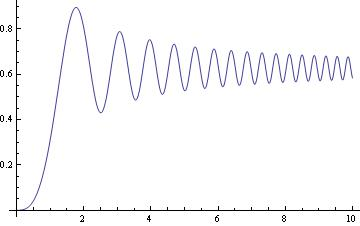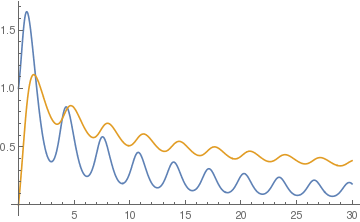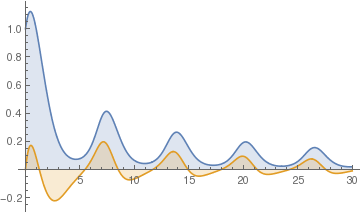# Preface

This section demontrates applications of standard Mathematica command NDSolve for solving differential equations numerically. It will be widely used in other sections of this tutorial.

# Numerical Solutions

Although sometimes Mathematica is able to solve a differential equation, in practice we tend to rely on numerical methods to solve differential equations. Most differential equations that are found in actual practice are much too complicated to express a solution using elementary or special functions. Usually, we want to obtain a solution in implicit form, but unfortunately this only happens occasionally. It is important to note that because a differential equation does not have an explicit or implicit solution does not necessarily mean that the solution does not exist.

Since a differential equation contains an unbounded derivative operato, we do not expect to obtain numerically the general solution that includes arbitrary constants. Instead, we knock out one solution by considering the initial value problem (IVP for short)

$y' = f(x,y), \qquad y\left( x_0 \right) = y_0 ,$
which is assumed to have a unique solution. Here prime indicates differentiation with respect to x: $$y' = {\text d}y/{\text d}x .$$

Example: Consider the initial value problem $$y' = \sin \left( x^2 \right) , \quad y(0) =0 .$$ We try to find its solution using the standard Mathematica command DSolve:

exactsol = DSolve[{y'[x] == Sin[x^2], y == 0}, y[x], x]
Out= {{y[x] -> Sqrt[$Pi]/2] FresnelS[Sqrt[2/\[Pi]] x]}} Mathematica represents the solution through a special function, called the Fresnel integral: $$S(x) = \int_0^x \sin \left( t^2 \right) {\text d}t .$$ ?FresnelS FresnelS[z] gives the Fresnel integral S(z). >>Now we solve the same problem numerically using NDSolve. numsol = NDSolve[{y'[x] == Sin[x^2], y == 0}, y[x], {x, 0, 10}] Out= {{y[x] -> InterpolatingFunction[{{0., 10.}}],<>],[x]}} Plot[y[x] /. numsol, {x, 0, 10}, PlotRange -> All]Frensel integral. Mathematica code We calculate its approximate value at, say, x=6.5 as follows: numsol /. x -> 6.5 Out= {{y[6.5] -> 0.639918}} This indicates that y(6.5) approximately equal to 0.639918. We can improve accuracy by choosing the option PrecisionGoal. There are two main approaches to finding a numerical value for the solution to the initial value problem $$y' = f(x,y), \quad y( x_0 )= y_0 .$$ Its solution can be obtained using either DSolve (for solutions represented using known functions, if it is possible) or NDSolve (for numerical solutions). The first one is based on definition of the slope function f(x,y) as an expression. The second one (which is recommended) is to define the slope as a function. To see how it works, let us consider an example. A general approach for determing numerically solutions of the initial value problems and then plot them using Mathematica's capability is as follows. We demostrate all steps on the following initial value problem (IVP, for short): \[ y' \equiv \frac{{\text d}y}{{\text d}x} = \frac{1-x}{1+y^5}, \qquad y(0 ) = 1 ,.$To see a numerical value at, say x=3.0, we type: y[x] /. a /. x -> 3.0 Out= {-0.333563} a = NDSolve[{y'[x]== (1-x)/(1+y[x]^5),y==1}, y[x],{x,0,3}]; Plot[y[x] /. a, {x,0,3}] Since its solution is known in implicit form, there is an alternative way to plot the graph: psi[x_, y_] = y + 1/6 y^6 - x + 1/2 x^2 - 7/6; ContourPlot[psi[x, y] == 0, {x, 0, 2}, {y, -1, 3}, ContourLabels->True, ColorFunction->Hue] Contour plot. Mathematica code

The general reference for NDSolve applications is

Example: Consider the slope function f(x,y) = 1/(3*x - 2*y + 1). Then we find a numerical value treating f(x,y) as an expression.

fp = 1/(3*xinit - 2*y[xinit] + 1)
Out= 1/(1 + 3 xinit - 2 y[xinit])
f = DSolve[{y'[xinit] == fp, y == 0}, y, xinit]
Out= {{y -> Function[{xinit}, 1/6 (1 + 9 xinit - 2 ProductLog[1/2 E^(1/2 + (9 xinit)/2)])]}}
xinit = 1.2
Out= 1.2
yvalue = f[][][][]]       (* this is the value of the solution at the point x=1.2 *)
Out= 0.68101

Now we use the function DSolve to find the explicit solution:

DSolve[{y'[x] == 1/(3*x - 2*y[x] + 1), y == 0}, y[x], x]

Solve::ifun: Inverse functions are being used by Solve, so some solutions may not be found; use Reduce for complete solution information. >>
Out= {{y[x] -> 1/6 (1 + 9 x - 2 ProductLog[1/2 E^(1/2 + (9 x)/2)])}}
Now we define solution as a function of x:
f[x_] = y[x] /. %[]
Out= 1/6 (1 + 9 x - 2 ProductLog[1/2 E^(1/2 + (9 x)/2)])
f[1.2]
Out= 0.68101

Example: Consider the initial value problem (IVP) for the Riccati equation:

$\frac{{\text d}y}{{\text d} x} = x^2 - y^2 , \qquad y(0) = 1.$
Although Mathematica is able to solve this problems explicitly, its solution is represented through a special Bessel function.
DSolve[{y'[x] == x^2 - (y[x])^2 , y == 1}, y[x], x]
$y(x) = \frac{I_{1/4} \left( \frac{x^2}{2} \right)}{\Gamm \left( \frac{3}{4} \right)} .$
We present two other options to find a numerical value of a solution to the initial value problem using NDSolve.

NDSolve[{y'[x] == x^2 - (y[x])^2, y == 1}, y[x], {x, 0, 1.4}]
Out= {{y[x] -> InterpolatingFunction[{{0.,1.4}}, <>] [x]}}
fsol[x_] = y[x] /. %[]
Out= InterpolatingFunction[{{0.,1.4}}, <>] [x]
fsol[0.4]
Out= 0.732727
or
sol = NDSolve[{y'[x] == Sqrt[4*x + y[x] - 1], y == 1}, y, {x, 1, 3}]
y[x] /. sol /. x -> 3
Out= 7.456545294165829
The general reference for NDSolve applications is

As we see applications of NDSolve are very straightforward --- use the solution "interpolating function" as a regular function. When plotting the solution, Mathematica recommends to use the Evaluation. We give some examples.

Example: We consider the initial value problem for the oscillating slope function

$\frac{{\text d}y}{{\text d} x} = y\,\sin \left( 2x +y \right) , \qquad y(0) = 1.$First, we solve a first-order ordinary differential equation, which gives you decaying oscillations: sol1 = NDSolve[{y'[x] == y[x] Sin[2 x + y[x]], y == 1}, y, {x, 0, 30}]; Now we use it inside another equation. The important thing to remember is that the domain of the previous equations should be equal to or contain the domain of the next equations. sol2 = NDSolve[{z'[x]== -z[x]^2+First[Evaluate[y[x] /. sol1]], z == 0}, z, {x, 0, 30}] Then we plot both solutions. Indeed, first solution here plays the role of sort of a driving force, so on the graphs below you see synchronization between oscillations. Plot[{Evaluate[y[x] /. sol1], Evaluate[z[x] /. sol2]}, {x, 0, 30}, PlotRange -> All] Two solutions. Mathematica codeNow you can do more advanced things, like using also derivative of the "Interpolating Function" inside of new equations: sol3 = NDSolve[{y'[x] == y[x] Cos[x + y[x]], y == 1}, y, {x, 0, 30}]; sol4 = NDSolve[{z'[x]== -z[x]+First[y[x]/5 + y'[x] /. sol3], z == 0}, z, {x, 0, 30}]; Plot[{Evaluate[y[x] /. sol3], Evaluate[z[x] /. sol4]}, {x, 0, 30}, PlotRange -> All, Filling -> 0] The solution and its derivative. Mathematica code

■